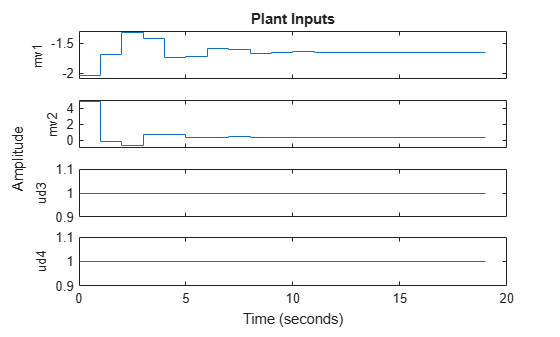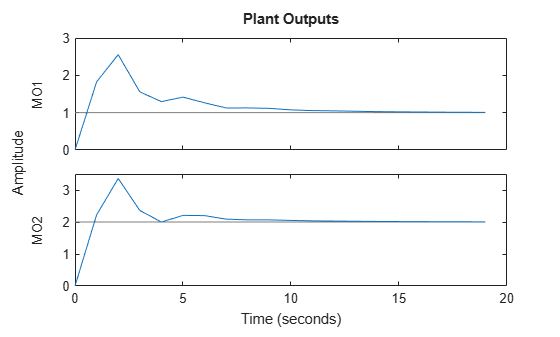# Simulating MPC Controller with Plant Model Mismatch

This example shows how to simulate a model predictive controller with a mismatch between the predictive plant model and the actual plant, as well as measured and unmeasured disturbances, using the `sim` command.

The predictive plant model has 2 manipulated variables, 2 unmeasured input disturbances, and 2 measured outputs. The actual plant has different dynamics.

### Define Plant Model

Define the parameters of the nominal plant which the MPC controller is based on. Systems from MV to MO and UD to MO are identical.

```p1 = tf(1,[1 2 1])*[1 1; 0 1]; plant = ss([p1 p1],'minimal'); plant.InputName = {'mv1','mv2','ud3','ud4'};```

### Design MPC Controller

Define inputs 1 and 2 as manipulated variables, 3 and 4 as unmeasured disturbances.

```plant = setmpcsignals(plant,'MV',[1 2],'UD',[3 4]); % Create the controller object with sampling period, prediction and control % horizons: mpcobj = mpc(plant,1,40,2);```
```-->The "Weights.ManipulatedVariables" property is empty. Assuming default 0.00000. -->The "Weights.ManipulatedVariablesRate" property is empty. Assuming default 0.10000. -->The "Weights.OutputVariables" property is empty. Assuming default 1.00000. ```

For unmeasured input disturbances, the MPC controller will use the following unmeasured disturbance model.

```distModel = eye(2,2)*ss(-.5,1,1,0); mpcobj.Model.Disturbance = distModel;```

### Define the Real Plant Model Used in Simulation

Define the parameters of the actual plant in closed loop with the MPC controller.

```p2 = tf(1.5,[0.1 1 2 1])*[1 1; 0 1]; psim = ss([p2 p2],'minimal'); psim = setmpcsignals(psim,'MV',[1 2],'UD',[3 4]);```

### Simulate Closed-Loop Response Using the SIM Command

Define reference trajectories and unmeasured disturbances entering the actual plant.

```dist = ones(1,2); % unmeasured disturbance signal refs = [1 2]; % output reference signal Tf = 20; % total number of simulation steps```

Create an MPC simulation options object. This allows you to define both unmeasured disturbances and a plant different than the one which the MPC controller uses as a prediction model.

```options = mpcsimopt(mpcobj); options.unmeas = dist; % unmeasured disturbance signal options.model = psim; % real plant model```

Run the closed-loop MPC simulation with model mismatch and unforeseen unmeasured disturbance inputs.

`sim(mpcobj,Tf,refs,options);`
```-->Converting model to discrete time. -->Assuming output disturbance added to measured output channel #1 is integrated white noise. -->Assuming output disturbance added to measured output channel #2 is integrated white noise. -->The "Model.Noise" property is empty. Assuming white noise on each measured output. -->Converting model to discrete time. ```The closed loop tracking performance is acceptable despite the presence of model mismatches and unmeasured input disturbances.